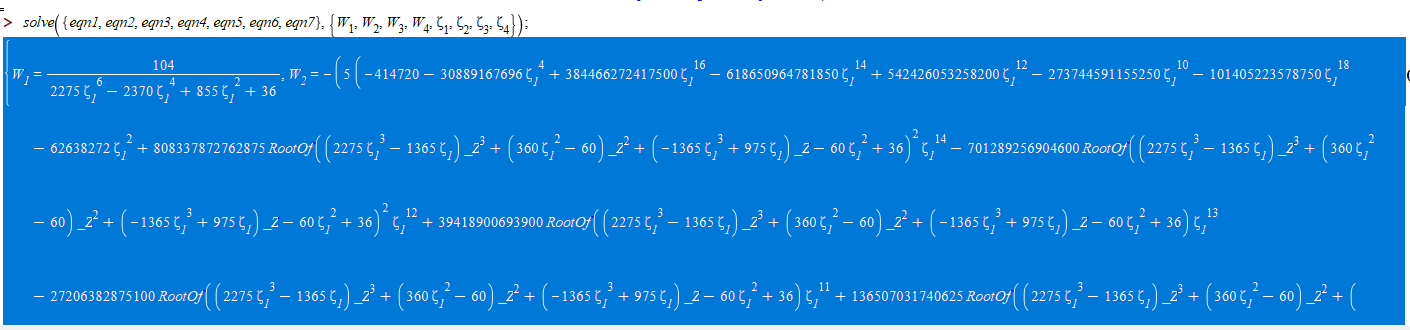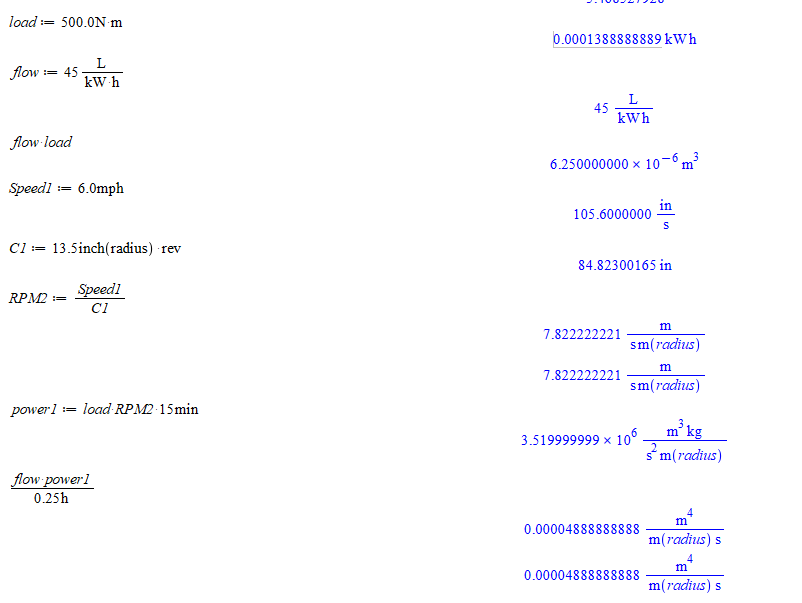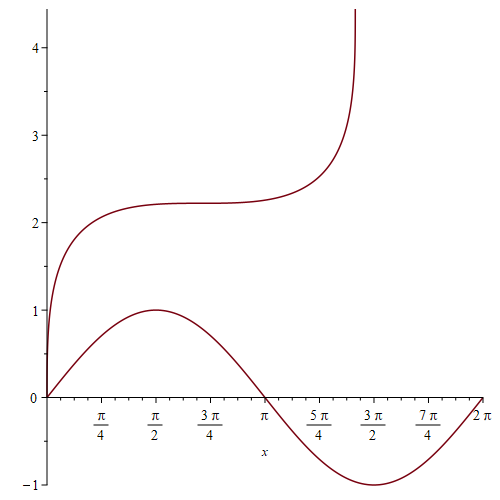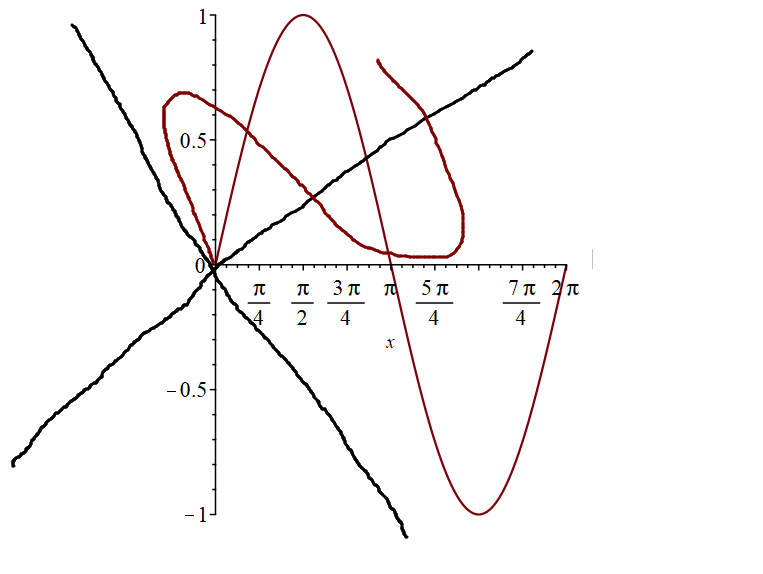## Kernel and image of a linear application...

I try to find kernel and image of a application whose i know the matrix.
restart;
with(LinearAlgebra);
A := Matrix([[1, 1, 1, -1], [-1, 1, -1, -1], [1, -1, -1, -1], [-1, -1, 1, 3]]);
k := op(NullSpace(A));#kernel
MatrixVectorMultiply(A, k);#check
C := op(ColumnSpace(A));
X := <x, y, z, t>;
F := MatrixVectorMultiply(A, X) - a*C - b*C - c*C;
G := op(convert(F, list));
solve({seq(G[i] = 0, i = 1 .. 4)}, {a, b, c}); why there is no solution ? Thank you.

## fprintf plot into a docx file...

How to fprintf say a plot into a docx file in maplesoft

kind help

## Solving a set of non-linear equations returned a w...

I'm solving a set of non-linear equations:

```eqn1 := W__1 + W__2 + W__3 + W__4 = 4;
eqn1 := W__1 + W__2 + W__3 + W__4 = 4

eqn2 := W__1*zeta__1 + W__2*zeta__2 + W__3*zeta__3 + W__4*zeta__4 = 0;
eqn2 := W__1 zeta__1 + W__2 zeta__2 + W__3 zeta__3 + W__4 zeta__4 =

0

eqn3 := W__1*zeta__1^2 + W__2*zeta__2^2 + W__3*zeta__3^2 + W__4*zeta__4^2 = 2/3;
2               2               2
eqn3 := W__1 zeta__1  + W__2 zeta__2  + W__3 zeta__3

2   2
+ W__4 zeta__4  = -
3

eqn4 := W__1*zeta__1^3 + W__2*zeta__2^3 + W__3*zeta__3^3 + W__4*zeta__4^3 = 0;
3               3               3
eqn4 := W__1 zeta__1  + W__2 zeta__2  + W__3 zeta__3

3
+ W__4 zeta__4  = 0

eqn5 := W__1*zeta__1^4 + W__2*zeta__2^4 + W__3*zeta__3^4 + W__4*zeta__4^4 = 2/5;
4               4               4
eqn5 := W__1 zeta__1  + W__2 zeta__2  + W__3 zeta__3

4   2
+ W__4 zeta__4  = -
5

eqn6 := W__1*zeta__1^5 + W__2*zeta__2^5 + W__3*zeta__3^5 + W__4*zeta__4^5 = 0;
5               5               5
eqn6 := W__1 zeta__1  + W__2 zeta__2  + W__3 zeta__3

5
+ W__4 zeta__4  = 0

eqn7 := W__1*zeta__1^6 + W__2*zeta__2^6 + W__3*zeta__3^6 + W__4*zeta__4^6 = 2/7;
6               6               6
eqn7 := W__1 zeta__1  + W__2 zeta__2  + W__3 zeta__3

6   2
+ W__4 zeta__4  = -
7

eqn8 := W__1*zeta__1^7 + W__2*zeta__2^7 + W__3*zeta__3^7 + W__4*zeta__4^7 = 0;
7               7               7
eqn8 := W__1 zeta__1  + W__2 zeta__2  + W__3 zeta__3

7
+ W__4 zeta__4  = 0

solve({eqn1, eqn2, eqn3, eqn4, eqn5, eqn6, eqn7}, {W__1, W__2, W__3, W__4, zeta__1, zeta__2, zeta__3, zeta__4});
```

However, the result looks like this:How should I obtain numerical values for W1,W2,W3,W4,zeta1,zeta2,zeta3,zeta4 ?

## How to pass in temporary values to parameters of a...

I have an array with a list of parameters. Suppose there are three parameters, t1, t2 and t3. I like to evaulate the array for different values of the parameters. I can do it manually by a command such as

eval(array, {t1=1, t2=5, t3=10})

What is a more systematic approach and how can it be done? Suppose I do not know the number of paramters in advance and the number of parameters could possible be large. Once I know the number of parameters, I would like to map a list of values to the parameters.

## Excel arctan2 ?...

Is there any equivalent to Excel function arctan2?

This function takes x and y values, and returns values from -Pi to +Pi. In that way one can easily get the x and y values of an angle with correct signs.

## Physics package problem with Define command...

Hello,

I have a problem regarding the physics package, which concerns, more specifically, the Define command.

It seems to be the case that it provides the wrong answer, and blatantly so, and I can't see what I am doing wrong for the life of me.

I want to build a fast way of doing coordinate transformations in general relativity, for which the basis vectors are called E[mu,~nu]. XX[~alpha] is the old coordinates expressed in terms of the new (in this case, the spherical coordinates are expressed in terms of cylindrical ones, i.e. [t,r, theta, phi]->[t, rho, phi,z].) As can be seen, only the first component in XX depends on time, but E contains weird elements (look at, for example, E[1,~2].) But when I do the calculation manually, it returns the expected results.What am I doing wrong? I really appreciate any help you can provide.

Benzema

## Plotting multiple items...

Are there any examples showing how to plot multiple items in one plot?

• What I have started with is to put a Plot component in my sheet, so that I can use that by its name.
• Next thing on the list was to generate different plot objects, by assigning each of them to a variable.
• To plot the whole bunch, I collected all the items I want to plot in a list
• And plotted them by SetProperty("MyPlotcomponent", value, display(plotlist))

I wonder if this is the best way to do it. Especially when adding more stuff afterwards, would it be possible to just add something to the plot component?

Do I need plot components at all?

## Copy values of mutable content...

What's the correct way of copying the current contents of a mutable object to a variable.

Apparently eval doesn't do the job.(1)(2)(3)
 >(4)(5)(6)>(7)## the error in summation...

the error in summation , How can this be corrected?

restart;

U := x^2;

for k from 0 to 1 do U[k+1] := sum(U[s]*(diff(U[k-s], x)), s = 0 .. k) end do;
U*(diff(U, x));
3
2 x
U;
0

## problems with revolutions, radians, RPM Units, rot...

simplified_MPH_to_RPM_to_engineering_units.mw

Good Morning,

I don't know why I have such difficulties with rotational units, esp the 2*pi conversions with radians and revolutions.

We are often back solving from MPH of the vehicle back to RPM of the wheel (revolutions per minute [or seconds]) to Rad/sec for engineering units such as torque*RPM calcs for power - kW.

Almost everytime I try to use units to convert the units the worksheet will revert to "atomic" units.   I quote "atomic" as this is what I appears to be.:  See graphic (also simplifiied worksheet attached)

The unit length(radius) seems to be a factor that is not well documented.   It also seems the earlier rplies include extra work to use convert and possibly "symbolic = true") as an extra step per Acer in an earlier posting several years ago.

When running calcs the convert, or simplify (to cancel length units m and inches out) the cancelations and the unit combinations seem to work awkwardly in this particular area.## How to integrate this expression?...

Let

```f := beta/(2*sigma*GAMMA(1/beta))*exp(-(abs(x-mu)/sigma)^beta);
```

where mu::real, beta > 0, sigma > 0.
How can we obtain the expression of F?

`F := int(f, x=-infinity..s)`

Reverse problem (a priori simpler):
It's known that

`F := 1/2+signum(x-mu)/(2*GAMMA(1/beta))*(GAMMA(1/beta)-GAMMA(1/beta, abs((x-mu)/sigma)^beta))`

How can we check that diff(F, x)=f?
Even with the assumptions on beta, mu and sigma and additional assumptions x>0 or x<0, I can't verify that diff(F, x)=f.

## How I solve PDE 1-D Wave Equation with initial and...

Hello, dear members! I try to solve the 1-D Wave Equation with initial and boundary conditions. And I have a problem when I'm trying to find the coeffs of Fourier series, that they depend on initial conditions. This is my code for Maple:

```restart;
with(PDETools);

# set the PDE, ic(initial conds) and bc (boundary conds)
pde := diff(u(x, t), t, t) = a^2*diff(u(x, t), x, x);
ic := u(x, 0) = 0, D(u)(x, 0) = psi(x);
bc := u(0, t) = 0, u(l, t) = 0;
psi := piecewise(0 <= x and x <= l/2, x, l/2 <= x and x <= l, l - x);

# using Fourier method for solving (I require use this methods)
res := pdsolve(pde, HINT = T(t)*X(x));

ode1 := op(1, op(1, op(2, res)));

ode2 := op(2, op(1, op(2, res)));

ode2 := subs(_c = -lambda, ode2);

# solve 2 ODE
# equation rely X(x)
dsolve({ode2, X(0) = 0}, X(x));

sin(sqrt(lambda)*l) = 0;

solve(%, lambda, allsolutions);

lambda := (Pi*n/l)^2;

X := (x, n) -> sin(Pi*n*x/l);

# equation rely T(t)
ode1 := subs(_c = -lambda, ode1);

dsolve({%, T(0) = 0}, T(t));

T := (t, n) -> C1[n]*sin(Pi*n*a*t/l);

# write the general solutions
Un := (x, t, n) -> T(t, n)*X(x, n);

# find the coeffs series
simplify(subs(t = 0, diff(u(x, t), t)) = psi);

((2/l)*l/(Pi*n*a)*int(psi*X(x, n), x = 0 .. l) assuming (x < l))
simplify(%) assuming n::posint;
combine(%);
C1[n] := factor(%)
```

But I got it:How to find this C1 coefficient and solve this equation? Maple doesn't work now. This command does not give the result.
I attached mws files, so pdeLR_11.mw is another code for solving, but it is not working so((!

Maybe I use this command wrong, or step-function is not convenient for maple?

## How do I rotate the axes the same Angle as the ima...

I know that the "rotate" can rotate image of a function, but we see that the coordinate axes don't change as much. For example:

with(plots):
with(plottools):
p:=plot(sin(x),x=0..2*Pi):
r := rotate(p, Pi/4):display(p, r)Although someone seems to have asked a similar question a long time ago, it was far from what I needed.

https://www.mapleprimes.com/questions/97812-Flip-Plot-Exchange-X-And-Yaxes

What I want to do is rotate the axes and the image at the same time rather than just flip axes.  The schematic diagram is as follows.## the error when drawing...

How can the value of uu(.1, .1, .1)  as a number, and what is the error when drawing?

restart;
uu := proc (x, y, t) options operator, arrow; e^(.2*t)*(e^(-x)+e^(-y)) end proc;

evalf(uu(.1, .1, .1));
2.
-----
0.08
e
plot3d(uu(x, y, .1), x = 1 .. 2, y = 1 .. 2);
Warning, expecting only range variables [x, y] in expression e^.2e-1*(e^(-x)+e^(-y)) to be plotted but found name e